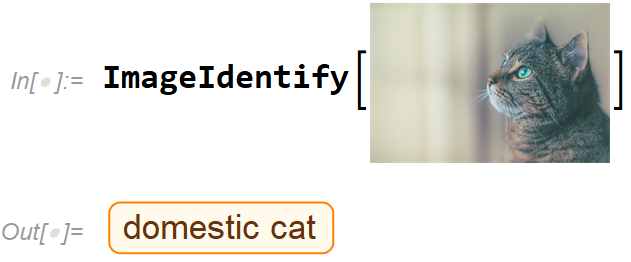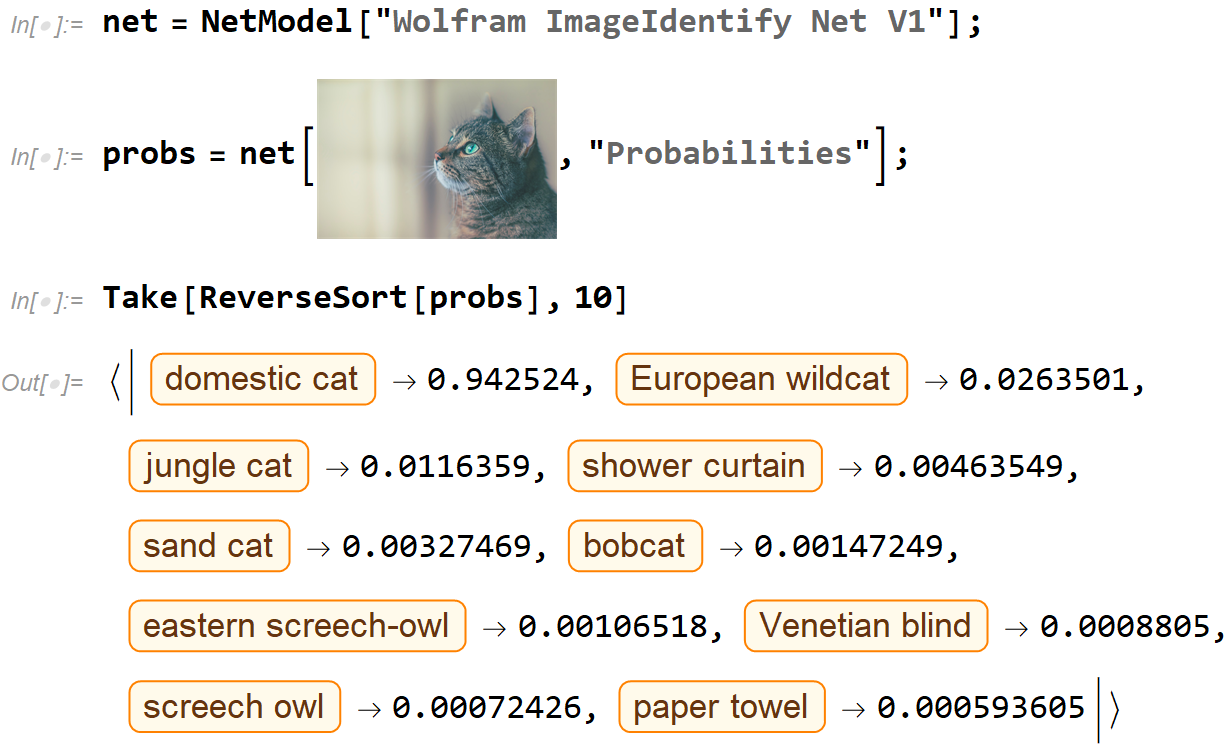# Exploring the Softmax FunctionExploring the Softmax Function. Developing Intuition With the Wolfram Language

In machine learning, classification problems are often solved with neural networks which give probabilities for each class or type it is trained to recognize. A typical example is image classification where the input to a neural network is an image and the output is a list of possible things that image represents with probabilities.

The Wolfram Language (WL) comes with a large library of pre-trained neural networks including ones that solve classification problems. For example, the built-in system function ImageIdentify uses a pre-trained network that can recognize over 4,000 objects in images.Image by the author using a photo by James Sutton on Unsplash

Side note: Because of the unique typesetting capabilities of the Wolfram notebook interface (such as mixing code with images), all code is shown with screen captures. A notebook with full code is included at the end of this story.

You can use the underlying neural network directly to access the probabilities for each of the 4,000+ possible objects. Clearly “domestic cat” wins hands down in this case with a probability of almost 1. Other types of cat follow with lower probabilities. The result for “shower curtain” is probably because of the background of the image. Summing up all the 4,000+ probabilities gives the number 1.0.## Fundamentals of Neural Network in Machine Learning

Fundamentals of Neural Network in Machine Learning. What is a Neuron? What is the Activation Function? How do Neural Network Works? How do Neural Networks Learn?

## What is Supervised Machine Learning

What is neuron analysis of a machine? Learn machine learning by designing Robotics algorithm. Click here for best machine learning course models with AI

## Pros and Cons of Machine Learning Language

AI, Machine learning, as its title defines, is involved as a process to make the machine operate a task automatically to know more join CETPA

## Quantum Machine Learning: learning on neural networks

Quantum Machine Learning: learning on neural networks. Analytical gradient computation, the Hadamard test, and more. This time, we’re going a little deeper into the rabbit hole and looking at how to build a neural network on a quantum computer.

## Artificial Neural Networks — Recurrent Neural Networks

Artificial Neural Networks — Recurrent Neural Networks. Remembering the history and predicting the future with neural networks. A intuition behind Recurrent neural networks.